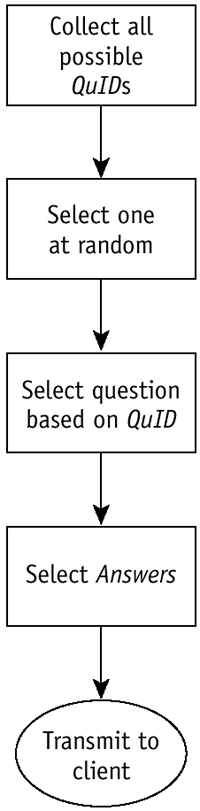# Scalable Alternative

Another possibility is that we can just get all the questions and set the internal pointer to a random row. While it does require a large space for storing the result, this solution will work better if our QuID s have a lot of holes, if questions are often deleted, or if they are entered in nonsequential order. The code necessary follows the logic in Figure 13.3 and looks like this:

##### Figure 13.3. Alternative Linear QuID Selection Method##### PHP
` //The only portion of this code that changed was the constructor function Question( ) {   MySQL_connect('sql.useractive.com', 'bigfun', '[REDACTED]');   MySQL_select_db('bigfun');   \$res = MySQL_query ("select QuID from Questions" );   \$WhichRow = rand(0,MySQL_num_rows(\$res)-1);   \$WhichQuid = MySQL_result(\$res,\$WhichRow,0);   \$res = MySQL_query ("select * from Questions where QuID = \$WhichQuid" );   \$sqlquestion = MySQL_fetch_array (\$res );   \$sqlQuid = \$sqlquestion["QuID"];   \$this->Q = \$sqlquestion["Q"];   \$this->idkey= \$sqlquestion["QuID"];   MySQL_free_result(\$res);   \$res = MySQL_query ("select * from Answers where WhichQuestion =          \$sqlQUid");   \$numrows = MySQL_num_rows(\$res);   for(\$i=0;\$i<\$numrows;\$i++) {    \$temp = MySQL_fetch_array(\$res);    array_push (\$this->A , \$temp["AnswerChoice"]);   } return \$this; //     } `

No loop, cleaner flowchart.

Only a little bit of the code was changed, the part that selected the QuID used.

##### PHP
` \$res = MySQL_query ("select QuID from Questions" ); `

Get all the QuID s of all the questions. Load them all into RAM.

##### PHP
` \$WhichRow = rand(0,MySQL_num_rows(\$res)-1); `

Choose a random row of data. The rows are contiguous and zero-based . There are MySQL_num_rows(\$res) of them. The bounds on rand are inclusive.

##### PHP
` \$WhichQuid = MySQL_result(\$res,\$WhichRow,0); `

This code selects a field; specifically , it selects the QuID in the randomly selected row.

##### PHP
` \$res = MySQL_query ("select * from Questions where QuID = \$WhichQuid" ); `

This code retrieves the information about the Question whose QuID we have chosen . The rest of the code is the same between the two versions.Flash and XML[c] A Developer[ap]s Guide
ISBN: 201729202
EAN: N/A
Year: 2005
Pages: 160